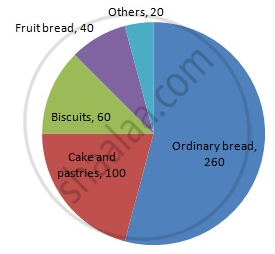Share

# In One Day the Sales (In Rupees) of Different Items of a Baker'S Shop Are Given Below: - Mathematics

Course
ConceptCircle Graph Or Pie Chart - Drawing Pie Charts

#### Question

In one day the sales (in rupees) of different items of a baker's shop are given below:

 Items Ordinary bread Fruit bread Cakes and Pastries Biscuits Others Total Sales (in Rs) 260 40 100 60 20 480

Draw a pie-chart representing the above sales.

#### Solution

We know:
Central angle of a component = (component value/sum of component values x 360)
Here, total sales = Rs 480
Thus, the central angle for each component can be calculated as follows:

 Item Sale (in Rs) Sector angle Ordinary bread 260 260/480 x 360 = 195 Fruit bread 40 40/480 x 360 = 30 Cakes and pastries 100 100/480 x 360 = 75 Biscuits 60 60/480 x 360 = 45 Others 20 20/480 x 360 = 15

Now, the pie chat representing the given data can be constructed by following the steps below:
Step 1 : Draw circle of an appropriate radius.
Step 2 : Draw a vertical radius of the circle drawn in step 1.
Step 3 : Choose the largest central angle. Here, the largest central angle is 195o. Draw a sector with the central angle 195o in such a way that one of its radii coincides with the radius drawn in step 2 and another radius is in its counter clockwise direction.
Step 4 : Construct other sectors representing other items in the clockwise direction in the descending order of magnitudes of their central angles.
​Step 5 : Shade the sectors with different colours and label them, as shown as in the figure below.​​Is there an error in this question or solution?

#### APPEARS IN

RD Sharma Solution for Mathematics for Class 8 by R D Sharma (2019-2020 Session) (2017 to Current)
Chapter 25: Data Handling-III (Pictorial Representation of Data as Pie Charts or Circle Graphs)
Ex. 25.1 | Q: 3 | Page no. 12

#### Video TutorialsVIEW ALL 

Solution In One Day the Sales (In Rupees) of Different Items of a Baker'S Shop Are Given Below: Concept: Circle Graph Or Pie Chart - Drawing Pie Charts.
S